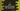# How to print a pascal's triangle in C with explanation# Pascal’s triangle in C program:

Pascal’s triangle is a triangle where each entry is the sum of the two numbers directly above it. This is a symmetric triangle, i.e. the left side numbers are identical to the right side numbers. Below is a pascal’s triangle of height 10 :

``````                             1
1     1
1     2     1
1     3     3     1
1     4     6     4     1
1     5    10    10     5     1
1     6    15    20    15     6     1
1     7    21    35    35    21     7     1
1     8    28    56    70    56    28     8     1
1     9    36    84   126   126    84    36     9     1``````

In this tutorial, we will learn how to print Pascal’s triangle in C program. The program will take the height as an input from the user and print it out.

## Algorithm to print it :

It looks complex, but if you do understand the algorithm properly, you can write a program. Let’s divide the problem :

• We need to print numbers
• We need to print blank spaces

Consider one small triangle of height 5 :

``````      	 1
1   1
1   2   1
1   3   3   1
1   4   6   4   1``````

If we print * instead of blank space, it will look like :

``````****1
***1*1
**1*2*1
*1*3*3*1
1*4*6*4*1``````

Here, height = 5

• for row = 1, total number of elements = 5, number is printed on position 5
• for row = 2, total number of elements = 6, number is printed on position 4,6
• for row = 3, total number of elements = 7, number is printed on position 3,5,7
• for row = 4, total number of elements = 8, number is printed on position 2,4,6,8
• for row = 5, total number of elements = 5, number is printed on position 1,3,5,7,9

so,

• The program will start i from 1 to rows
• j will run from 1 to i + rows - 1
• If the total number of elements is odd, the numbers are also odd. Else these are even.
• If the value of j is greater than or equal to rows - i, we are starting to print numbers.
• One blank space is printed between two numbers.

The nth entry of Pascal’s triangle for row is :

``row! / (n! * (row - n)!);``

## C program :

The complete C program to print the pascal triangle is as below :

``````#include <stdio.h>
long findFactorial(long);
long findEntry(long, long);
void printEntry(long);

int main()
{
long rows;
long i, j, current;
long entry;

printf("Enter the number of rows : \n");
scanf("%ld", &rows);

for (i = 1; i <= rows; i++)
{
current = 0;
for (j = 1; j <= i + rows - 1; j++) { if ((i + rows - 1) % 2 != 0) { if (j >= (rows - i) && j % 2 != 0)
{
printEntry(findEntry(i - 1, current));
current++;
}
else
{
printf("   ");
}
}
else
{
if (j >= (rows - i) && j % 2 == 0)
{
printEntry(findEntry(i - 1, current));
current++;
}
else
{
printf("   ");
}
}
}
printf("\n");
}
return 0;
}

void printEntry(long value)
{
if (value < 10)
{
printf("  %ld", value);
}
else if (value < 100)
{
printf(" %ld", value);
}
else
{
printf("%ld", value);
}
}

long findEntry(long row, long position)
{
return findFactorial(row) / (findFactorial(position) * findFactorial(row - position));
}

long findFactorial(long n)
{
if (n == 0)
{
return 1;
}
else
{
return n * findFactorial(n - 1);
}
}``````

### Sample Output :

``````Enter the number of rows :
5
1
1     1
1     2     1
1     3     3     1
1     4     6     4     1

Enter the number of rows :
10
1
1     1
1     2     1
1     3     3     1
1     4     6     4     1
1     5    10    10     5     1
1     6    15    20    15     6     1
1     7    21    35    35    21     7     1
1     8    28    56    70    56    28     8     1
1     9    36    84   126   126    84    36     9     1

Enter the number of rows :
15
1
1     1
1     2     1
1     3     3     1
1     4     6     4     1
1     5    10    10     5     1
1     6    15    20    15     6     1
1     7    21    35    35    21     7     1
1     8    28    56    70    56    28     8     1
1     9    36    84   126   126    84    36     9     1
1    10    45   120   210   252   210   120    45    10     1
1    11    55   165   330   462   462   330   165    55    11     1
1    12    66   220   495   792   924   792   495   220    66    12     1
1    13    78   286   715   1287   1716   1716   1287   715   286    78    13     1
1    14    91   364   1001   2002   3003   3432   3003   2002   1001   364    91    14     1``````

Note that the triangle will not print properly if the height is very high. We are using long and it will overflow the length of long.